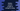# Python program to convert a tuple of strings to string## Python program to convert a tuple of strings to string:

In this post, we will learn how to convert a tuple of strings to a single string in Python. I will show you two different ways to do that. We can use this method to convert a tuple of characters to a string.

Let’s see how to do that with examples:

## Method 1: By using str.join():

join() is the easiest way to convert a tuple to string. We can pass one separator to str.join(). If we provide a separator, it will use that separator to separate each strings while joining.

Let’s see how to do this with an example:

``````def tuple_to_str(t):
return ''.join(t)

given_tuple = ('hello', 'world')

print(tuple_to_str(given_tuple))``````

Here,

• tuple_to_str method is used to convert a tuple to a string. It is taking a tuple as parameter and returns one string by joining all strings using join.

If you run this program, it will print the below output:

``helloworld``

### How to use a different separator:

We can also use as different separator like a comma ,. For example, the below program uses a comma to separate all words:

``````def tuple_to_str(t):
return ','.join(t)

given_tuple = ('hello', 'world')

print(tuple_to_str(given_tuple))``````

This program will use a comma as the separator. If you run this program, it will print:

``hello,world``

## Method 2: By using a loop:

We can also use a loop to iterate through the items and append them to a string. We can use a for-in loop for that:

``````def tuple_to_str(t):
result = ''
for str in t:
result += str
return result

given_tuple = ('hello', 'world')

print(tuple_to_str(given_tuple))``````

Here,

• result is an empty string.
• We are using a for…in loop to iterate through the strings in the tuple. For each string, we are adding it to the result string.
• It returns result.

If you run this program, it will print:

``helloworld``

### Conclusion:

Both str.join and loop gives the same result. join is easier to use and we can also define a separator. So, join is preferred over any other way to convert a tuple of strings to a string.